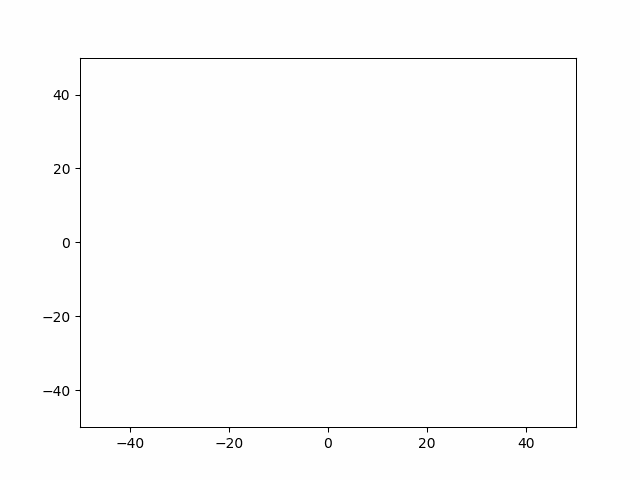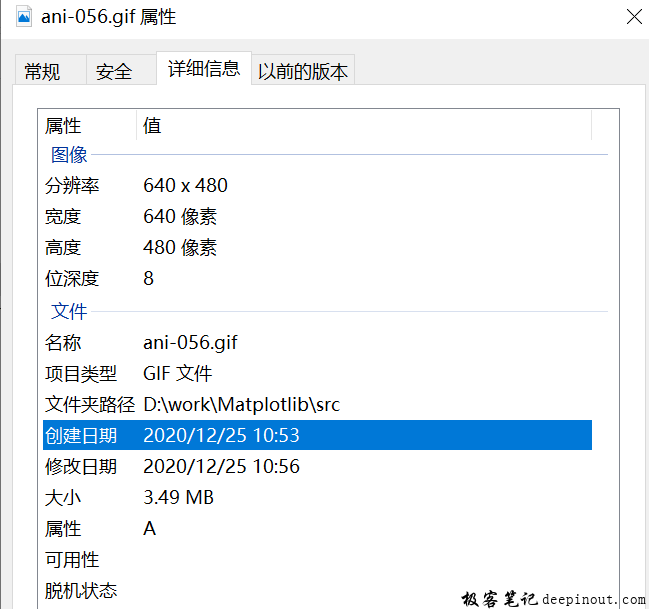# Matplotlib 输出gif文件`pw_writer = animation.PillowWriter(fps = 20)`

`anim.save('ani-056.gif', writer = pw_writer)`

``````import numpy as np
from matplotlib import pyplot as plt
from matplotlib import animation

# 创建绘图轴对象，创建一个空白窗口
fig = plt.figure()
axis = plt.axes(xlim =(-50, 50), ylim =(-50, 50))
line, = axis.plot([], [], lw = 2)

#创建全局数据保存列表
xdata, ydata = [], []

#定义每次重复清空函数
def init():
global xdata, ydata
xdata, ydata = [], []
line.set_data([], [])
return line,

#每帧动画更新函数
def animate(i):
# t 帧序号
t = 0.1 * i

# x, y 绘图
x = t * np.sin(t)
y = t * np.cos(t)

# 增加到列表，连接起所有点。
xdata.append(x)
ydata.append(y)
line.set_data(xdata, ydata)

return line,

anim = animation.FuncAnimation(fig, animate, init_func = init,
frames = 500, interval = 20, blit = True)

#保存的动画文件名为当前文件夹下的ani-056.gif，帧率为20帧每秒，格式为gif。
pw_writer = animation.PillowWriter(fps = 20)
anim.save('ani-056.gif', writer = pw_writer)

plt.show()  # 会一直循环播放动画
``````

• 微信订阅
• 回顶